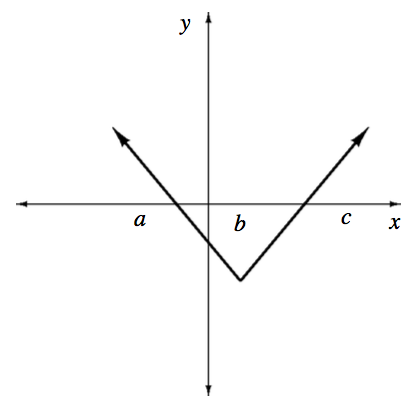### Home > APCALC > Chapter 8 > Lesson 8.2.1 > Problem8-73

8-73.Multiple Choice: The function $f$, whose graph consists of two line segments, is shown at right. Which of the following are true for $f$ on the open interval $(a,c)$?

1. The domain of the derivative of $f$ is the open interval $(a,c)$.

2. $f$ is continuous on the open interval $(a,c)$.

3. The derivative of $f$ is positive on the open interval $(b,c)$.

1. I only

1. II only

1. III only

1. II & III only

1. I, II, & III

Is there anywhere within $a in which the derivative does not exist?

Check all three conditions of continuity.

A positive derivative means that $f(x)$ is increasing.